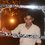# What's wrong here??

In how many ways the letters of the word "INSURANCE" be arranged so that the vowels never occur together?

This is how I did the problem,

Since number of permutations of n different things taken all at a time, when m specified things come together is $(n!-m!)\times (n-m+1)!$

Number of ways the vowels never occur together = $(9!-4!)\times (9-4+1)!$

which gives a value greater than the total number of permutations with that word!!!

How is it possible?Is the formula wrong?

Another way is to find answer is,

Number of ways vowels never occur together=Total permutations - No.of permutations in which vowels occur together =$\frac { 9! }{ 2! } -\frac { 6!\times 4! }{ 2! } =172800$

Is my second way correct?Note by Anandhu Raj
5 years, 3 months ago

This discussion board is a place to discuss our Daily Challenges and the math and science related to those challenges. Explanations are more than just a solution — they should explain the steps and thinking strategies that you used to obtain the solution. Comments should further the discussion of math and science.

When posting on Brilliant:

• Use the emojis to react to an explanation, whether you're congratulating a job well done , or just really confused .
• Ask specific questions about the challenge or the steps in somebody's explanation. Well-posed questions can add a lot to the discussion, but posting "I don't understand!" doesn't help anyone.
• Try to contribute something new to the discussion, whether it is an extension, generalization or other idea related to the challenge.

MarkdownAppears as
*italics* or _italics_ italics
**bold** or __bold__ bold
- bulleted- list
• bulleted
• list
1. numbered2. list
1. numbered
2. list
Note: you must add a full line of space before and after lists for them to show up correctly
paragraph 1paragraph 2

paragraph 1

paragraph 2

[example link](https://brilliant.org)example link
> This is a quote
This is a quote
    # I indented these lines
# 4 spaces, and now they show
# up as a code block.

print "hello world"
# I indented these lines
# 4 spaces, and now they show
# up as a code block.

print "hello world"
MathAppears as
Remember to wrap math in $$ ... $$ or $ ... $ to ensure proper formatting.
2 \times 3 $2 \times 3$
2^{34} $2^{34}$
a_{i-1} $a_{i-1}$
\frac{2}{3} $\frac{2}{3}$
\sqrt{2} $\sqrt{2}$
\sum_{i=1}^3 $\sum_{i=1}^3$
\sin \theta $\sin \theta$
\boxed{123} $\boxed{123}$

Sort by:

Also it is not stated that n and m are all distinct or not if you use the formula you can't take n=9

- 5 years, 3 months ago

May be!!:)

- 5 years, 3 months ago

The Second way is unquestionably correct.

- 5 years, 3 months ago

What about the first one? Whether such a formula exist(seen it in a book)?

- 5 years, 3 months ago

No but if u have its a misprint.

- 5 years, 3 months ago

Thanks :)

- 5 years, 3 months ago